Sub­sec­tions

### D.37 Forces by par­ti­cle ex­change de­riva­tions

#### D.37.1 Clas­si­cal en­ergy min­i­miza­tion

The en­ergy min­i­miza­tion in­clud­ing a se­lec­ton is es­sen­tially the same as the one for only spo­ton and fo­ton field. That one has been dis­cussed in chap­ter A.22.1 and in de­tail in {A.2}. So only the key dif­fer­ences will be listed here.

The en­ergy to min­i­mize is now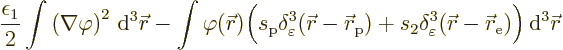So the only real dif­fer­ence in the vari­a­tional analy­sis is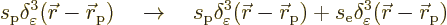That means that the Pois­son equa­tion now be­comes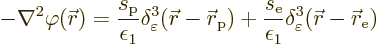Since the Pois­son equa­tion is lin­ear, the so­lu­tion is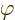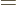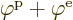. Here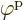is the fo­ton field (A.107) pro­duced by the spo­ton as be­fore, and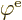is a sim­i­lar ex­pres­sion, but us­ing the se­lec­ton sarge and dis­tance from the se­lec­ton: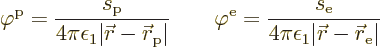The en­ergy low­er­ing is now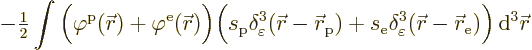Mul­ti­ply­ing out, you get, of course, the en­ergy low­er­ings for the spo­ton and se­lec­ton in iso­la­tion. But you also get two ad­di­tional in­ter­ac­tion terms be­tween these sarges. These two terms are equal; the se­lec­ton fieldeval­u­ated at the po­si­tion of the spo­ton times spo­ton sarge is the same as the spo­ton fieldat the se­lec­ton times se­lec­ton sarge. So it is seen that each term con­tributes half to the Koulomb en­ergy as claimed in the text.

The fo­ton field en­ergy is still half of the par­ti­cle-field in­ter­ac­tion en­er­gies and of op­po­site sign. That is why the en­ergy change is half of what you would ex­pect from the in­ter­ac­tion of the par­ti­cles with each other’s field: the other half is off­set by changes in field en­ergy.

#### D.37.2 Quan­tum en­ergy min­i­miza­tion

This de­riva­tion in­cludes the se­lec­ton in the spo­ton-fo­tons sys­tem an­a­lyzed in {A.22.3}. Since the analy­sis is es­sen­tially un­changed, only the key dif­fer­ences will be high­lighted.

If an se­lec­ton is added to the sys­tem, the sys­tem wave func­tion be­comes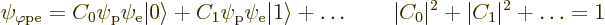The de­mon can hold the se­lec­ton in its other hand. The Hamil­ton­ian will now of course in­clude a term for the se­lec­ton in iso­la­tion, as well as an in­ter­ac­tion with the fo­ton field. These are com­pletely anal­o­gous to the cor­re­spond­ing spo­ton terms.

So the en­ergy to be min­i­mized for the ground state be­comes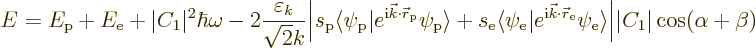If this is min­i­mized as in {A.22.3}, the en­ergy is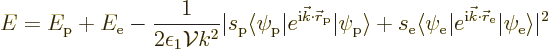The square ab­solute value of a quan­tity can be found as the prod­uct of that quan­tity times its com­plex con­ju­gate. That gives the same en­ergy low­er­ing as for the lone spo­ton, and a sim­i­lar term for a lone se­lec­ton. How­ever, there is an ad­di­tional term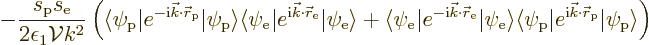If you write out the in­ner prod­uct in­te­grals over the se­lec­ton co­or­di­nates ex­plic­itly, this be­comes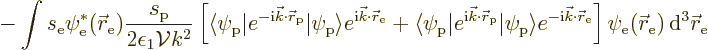Summed over all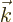, the sec­ond term in­side the square brack­ets gives the same an­swer as the first; that is be­cause op­po­siteval­ues ap­pear equally in the sum­ma­tion. Look­ing at the first term, the sum­ma­tion overpro­duces again the spo­ton po­ten­tial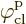, but now eval­u­ated at the po­si­tion of the se­lec­ton. That then shows the ad­di­tional en­ergy low­er­ing to be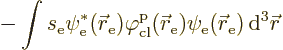Ex­cept for the dif­fer­ences in no­ta­tion, that is the same se­lec­ton-spo­ton in­ter­ac­tion en­ergy as found in {A.22.1}.

#### D.37.3 Rewrit­ing the La­grangian

The rules of en­gage­ment are as fol­lows:

• The Carte­sian axes are num­bered us­ing an in­dex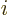, with1, 2, and 3 for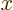,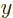, and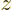re­spec­tively.
• Also,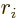in­di­cates the co­or­di­nate in thedi­rec­tion,,, or.
• De­riv­a­tives with re­spect to a co­or­di­nateare in­di­cated by a sim­ple sub­script.
• If the quan­tity be­ing dif­fer­en­ti­ated is a vec­tor, a comma is used to sep­a­rate the vec­tor in­dex from dif­fer­en­ti­a­tion ones.
• In­dex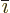is the num­ber im­me­di­ately fol­low­ingin the cyclic se­quence ...123123...and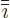is the num­ber im­me­di­ately pre­ced­ing.
• Ifis al­ready been used for some­thing else,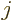can be used the same way.
• Time de­riv­a­tives are in­di­cated by a sub­script t.

Con­sider first the square mag­netic field: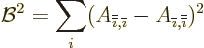Ex­pand­ing out the square, that is equiv­a­lent to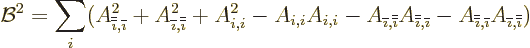The sum­ma­tion in­dices can now be cycli­cally re­de­fined to give an equiv­a­lent sum overequal to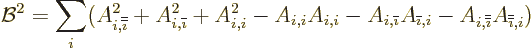The terms can be com­bined in sets of three as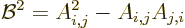Here sum­ma­tion overandis now un­der­stood.

The square elec­tric field is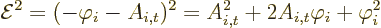All to­gether, that gives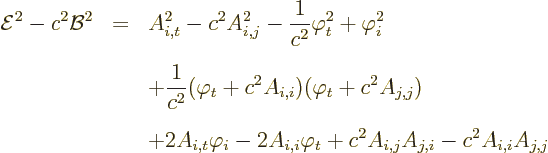as can be ver­i­fied by mul­ti­ply­ing out and sim­pli­fy­ing. The right hand side in the first line is the self-ev­i­dent elec­tro­mag­netic La­grangian den­sity, ex­cept for the fac­tor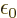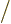​2. The sec­ond line is the square of the Lorentz con­di­tion quan­tity. The fi­nal line can be writ­ten as a sum of pure de­riv­a­tives: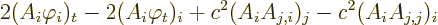Pure de­riv­a­tives do not pro­duce changes in the ac­tion, as the changes in the po­ten­tials dis­ap­pear on the bound­aries of in­te­gra­tion.

#### D.37.4 Coulomb po­ten­tial en­ergy

The Coulomb po­ten­tial en­ergy be­tween charged par­ti­cles is typ­i­cally de­rived in ba­sic physics. But it can also eas­ily be ver­i­fied from the con­ven­tional elec­tro­mag­netic en­ergy (A.143). In the steady case, there is only the elec­tric field, due to the Coulomb po­ten­tial. The en­ergy may then be writ­ten as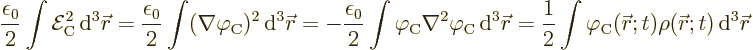where the first equal­ity comes from the de­f­i­n­i­tion of the elec­tric field, the sec­ond from in­te­gra­tion by parts and the third one from the first Maxwell equa­tion. Sub­sti­tu­tion of the Coulomb po­ten­tial in terms of the charge dis­tri­b­u­tion as given in {A.22.8},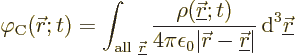now gives the Koulomb po­ten­tial en­ergy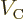for a con­tin­u­ous charge dis­tri­b­u­tion: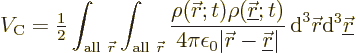For point charges, the charge dis­tri­b­u­tion is by de­f­i­n­i­tion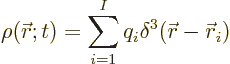Here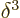is the three-di­men­sion­al delta func­tion,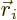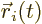the po­si­tion of point charge, and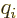its charge.

Re­call that the delta func­tion picks out the value atfrom what­ever it is in­te­grated against. Us­ing this twice on the Coulomb po­ten­tial en­ergy above,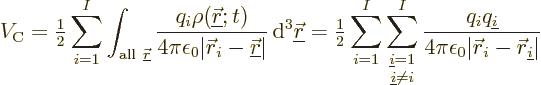That is the Coulomb po­ten­tial en­ergyfor point charges.

Note again that phys­i­cally all the en­ergy is in­side the elec­tro­mag­netic field. There is no en­ergy of in­ter­ac­tion of the charged par­ti­cles with the field. If equal charges move closer to­gether, they in­crease the en­ergy in the elec­tro­mag­netic field. That re­quires work.